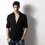Existence of Aliens - Pythagoras

Can anyone of you prove that Aliens doesn't exist using Pythagoras theorem. Actually it was asked by my companion. I didn't get it. I mean how the existence of Aliens can be proved by that theorem. If you guys know it then help me out.Note by Abhishek Kumar
6 years ago

MarkdownAppears as
*italics* or _italics_ italics
**bold** or __bold__ bold
- bulleted- list
• bulleted
• list
1. numbered2. list
1. numbered
2. list
Note: you must add a full line of space before and after lists for them to show up correctly
paragraph 1paragraph 2

paragraph 1

paragraph 2

[example link](https://brilliant.org)example link
> This is a quote
This is a quote
    # I indented these lines
# 4 spaces, and now they show
# up as a code block.

print "hello world"
# I indented these lines
# 4 spaces, and now they show
# up as a code block.

print "hello world"
MathAppears as
Remember to wrap math in $ ... $ or $ ... $ to ensure proper formatting.
2 \times 3 $2 \times 3$
2^{34} $2^{34}$
a_{i-1} $a_{i-1}$
\frac{2}{3} $\frac{2}{3}$
\sqrt{2} $\sqrt{2}$
\sum_{i=1}^3 $\sum_{i=1}^3$
\sin \theta $\sin \theta$
\boxed{123} $\boxed{123}$

Sort by:

It can be....Dave!...Although, I do not know how! I'm thinking, as there may be some contradiction in the theorem relating to aliens

- 6 years ago

as far as i searched....it was juz a proposal....nd that too not related to...E.T.'s(may be)

- 6 years ago

see a^2+b^2=c^2 this relation should hold for all values of a,b,c!!! As somewhere it does not hold therefor an ambiguity disposes off the 'EXISTENCE OF ALIEN"!!!

- 5 years, 11 months ago

Assume aliens exist Then given that there are at least two intelligent races in the universe, humanity is not a unique event and there must be an infinite number of intelligent species in the infinite universe. At least one of these races must have discovered Pythagoras' theorem before Pythagoras. If that was the case then the theorem would have been named after the alien discoverer. BUT the theorem is known as pythagoras' theorem which is a contradiction. Thus aliens do not exist. QED

- 6 years ago

I don't agree Aahitagni, may be aliens discovered it after humans or we don't know what they call it.

- 6 years ago

but there are an INFINITE number of aliens, so the probability of one of them discovering it before Humans is actually infinitesimally close to 1. The real flaw is the assumption that the theorem is named after the alien. How would we know that it is named after the alien?

- 6 years ago

Ya but we named it Pythagoras' Theorem in our world, and we consider Pythagoras to be the first person on earth, or we can say, the first person who we think actually existed, to have shown this theorem.

- 6 years ago

or may be Phytagoras is an allien that's make sense...lol

- 6 years ago

may be it was discovered before us named after someone else but it was not announced on earth

- 6 years ago

Then Aahitagni, you could also tell me that Aliens do not exist because Euclid was the first person to discover the Euclidian theorem...or Ramanujan was the first person to introduce a whole new set of theory of numbers... There's something else in the theorem that prompts us to think so....Preferably, I guess the right-angled triangle's vertices touching the circumference of a circle...maybe that way...I'm trying!

- 6 years ago

i didn't got ur xplaination......plzzz clarify....

- 6 years ago